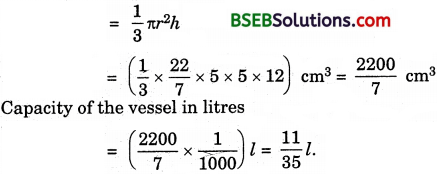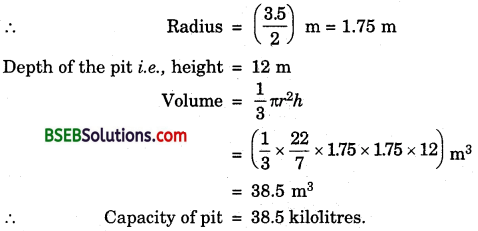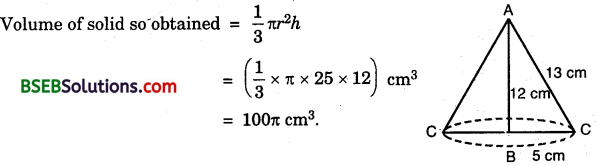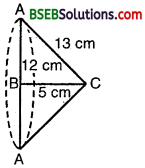# Bihar Board Class 9th Maths Solutions Chapter 13 Surface Areas and Volumes Ex 13.7

Bihar Board Class 9th Maths Solutions Chapter 13 Surface Areas and Volumes Ex 13.7 Textbook Questions and Answers.

## BSEB Bihar Board Class 9th Maths Solutions Chapter 13 Surface Areas and Volumes Ex 13.7Question 1.
Find the volume of the right circular cone with
(i) radius 6 cm, height 7 cm
(ii) radius 3.5 cm, height 12 cm
Solution:
(i) Here, r = 6 cm and h = 7 cm(ii) Here, r = 3.5 cm and h = 12 cmQuestion 2.
Find the capacity in litres with
(i) radius 7 cm, slant height 25 cm
(ii) height 12 cm, slant height 13 cm
Solution:
(i) Here, r = 7 cm and l = 25 cm.
Let the height of the cone be h cm. Then,
h² = l² – r² = 252 – 72
= 625 – 49 = 576
⇒ h = $$\sqrt{576}$$
= 24 cm
Volume of the conical vessel∴ Capacity of the vessel in litres = $$\left(\frac{1232}{1000}\right)$$l = 1.2321.

(ii) Here, h = 12 cm and l = 13 cm Let the radius of the base of the cone be r cm. Then,
r² = l² – h² = 13² – 12²
= 169 – 144 = 25
⇒ r = $$\sqrt{25}$$ = 5 cm
Volume of the conical vesselQuestion 3.
The height of a cone is 15 cm. If its volume is 1570 cm³, find the radius of the base. (Use π = 3.14)
Solution:
Here, h = 15 cm and volume = 1570 cm³ Let the radius of the base of cone be r cm.
Volume = 1570 cm³
⇒ $$\frac { 1 }{ 3 }$$πr²h = 1570
⇒ $$\frac { 1 }{ 3 }$$ x 3.14 x r² x 15 = 1570
⇒ r² = $$\frac{1570}{3.14 \times 5}$$ = 100
⇒ r = $$\sqrt{100}$$ = 10
Thus, the radius of the base of cone is 10 cm.

Question 4.
If the volume of a right circular cone of height 9 cm is 48 n cm³, find the diameter of its base.
Solution:
Here, h = 9 cm and volume = 48π cm³
Let the radius of the base of the cone be r cm
∴ Volume = 48n cm³
⇒ $$\frac { 1 }{ 3 }$$ πr²h = 48π
⇒ $$\frac { 1 }{ 3 }$$ x r² x 9 = 48π
⇒ 3r² = 48
⇒ r² = $$\frac{48}{3}$$ = 16
⇒ r = $$\sqrt{16}$$ = 4
Thus, the diameter of the base of the cone = 8 cm.Question 5.
A conical pit of top diameter 3.5 m is 12 m deep. What is its capacity in kilolitres?
Solution:
Diameter of the top of the conical pit = 3.5 mQuestion 6.
The volume of a right circular cone is 9856 cm³. If the diameter of the base is 28 cm, find
(i) height of the cone,
(ii) slant height of the cone,
(iii) curved surface area of the cone.
Solution:
(i) Diameter of the base of the cone = 28 cm
∴ Radius = r = ( $$\frac{28}{2}$$ ) cm = 14 cm.
Volume of the cone = 9856 cm³
Let the height of the cone be h cm.
Now, volume = 9856 cm³
⇒ $$\frac { 1 }{ 3 }$$ πr²h = 9856
⇒ $$\frac { 1 }{ 3 }$$ x $$\frac { 22 }{ 7 }$$ x 14 x 14 x h = 9856
⇒ h = $$\frac{9856 \times 3 \times 7}{22 \times 14 \times 14}$$ = 48
Thus, the height of the cone = 48 cm.

(ii) Here, r = 14 m and h = 48 cm
Let l be the slant height of the cone. Then,
l² = h² + r² = 48² + 14²
= 2304+ 196 = 2500
⇒ l = $$\sqrt{2500}$$ = 50
Thus, the slant height of the cone = 50 cm.

(iii) Here, r = 14 m and l = 50 cm.
Curved surface area = πrl
= ($$\frac { 22 }{ 7 }$$ x 14 x 50) cm²
= 2200 cm².Question 7.
A right triangle ABC with sides 5 cm, 12 cm and 13 cm is revolved about the side 12 cm. Find the volume of the solid so obtained.
Solution:
On revolving the right ∆ ABC about the side AB (= 12 cm), we get a cone as shown in the figure.Question 8.
If the triangle ABC in the question 7 above is revolved about the side 5 cm, then find the volume of the solid so obtained. Find also the ratio of the volumes of the two solids obtained in question 7 and 8.
Solution:
On revolving the right ∆ ABC about the side BC (= 5 cm), we get a cone as shown in the figure,Volume of solid so obtained = $$\frac { 1 }{ 3 }$$ πr²h
= $$\frac { 1 }{ 3 }$$ x π x 12 x 12 x 5 cm³
= 240π cm³
∴ Ratio of their volumes = 100π : 240π
(i.e., of Q 7 Q 8) = 5 : 12.Question 9.
A heap of wheat is in the form of a cone whose diameter is 10.5 m and height is 3 m. Find its volume. The heap is to be covered by canvas to protect it from rain. Find the area of the canvas required.
Solution:
Diameter of the base of the cone = 10.5 m
∴ Radius = r = $$\frac { 10.5 }{ 2 }$$ m = 5.25 m
Height of the cone = 3m
∴ Volume of the cone (heap) = $$\frac { 1 }{ 3 }$$πr²h
= ($$\frac { 1 }{ 3 }$$ x $$\frac { 22 }{ 7 }$$ x 5.25 x 5.25 x 3) m³
= 86.625 m³
To find the slant height l :
We have, l² = h² + r² = 3² + (5.25)²
= 9 + 27.5625 = 36.5625
⇒ l = $$\sqrt{36.5625}$$ = 6.0467 (approx.)
Canvas required to protect wheat from rain
= Curved surface area
= πrl = ($$\frac { 22 }{ 7 }$$ x 5.25 x 6.0467) m²
= 99.77 m² (approx.)